# State dependence and trajectory models

I am currently reviewing a paper that uses group based trajectory models (GBTM) – and to start this isn’t a critique of the paper. GBTM I think is a very useful descriptive tool (how this paper I am reading mostly uses it), and can be helpful in some predictive contexts as well.

It is much more difficult though to attribute a causal framework to those trajectories though. First, my favorite paper on this topic is Distinguishing facts and artifacts in group-based modeling (Skardhamar, 2010). Torbjørn in that paper simulates random data (not dissimilar to what I do here, but a few more complicated factors), and shows that purely random data will still result in GBTM identifying trajectories. You can go the other way as well, I have a blog post where I simulate actual latent trajectories and GBTM recovers them, and another example where fit stats clearly show a random effects continuous model is better for a different simulation. In real data though we don’t know the true model like these simulations, so we can only be reasonably skeptical that the trajectories we uncover really represent latent classes.

In particular, the paper I was reading is looking at a binary outcome, so you just observe a bunch of 0s and 1s over the time period. So given the limited domain, it is difficult to uncover really wild looking curves. They ended up finding a set of curves that although meet all the good fit stats, pretty much cover the domain of possibilities – one starting high an linearly sloping down, one starting low and sloping up, one flat high, one flat low, and a single curved up slope.

So often in criminology we interpret these latent trajectories as population heterogeneity – people on different curves are fundamentally different (e.g. Moffitt’s taxonomy for offending trajectories). But there are other underlying data generating processes that can result in similar trajectories – especially over a limited domain of 0/1 data.

Here I figured the underlying data the paper I am reviewing is subject to very strong state dependence – your value at t-1 is very strongly correlated to t. So here I simulate data in R, and use the flexmix package to fit the latent trajectories.

First, I simulate 1500 people over 15 time points. I assign them an original probability estimate uniformly, then I generate 15 0/1 observations, updating that probability slightly over time with an auto-correlation of 0.9. (Simulations are based on the logit scale, but then backed out into 0/1s.)

``````# R Code simulating state dependence 0/1
# data
library("flexmix")
set.seed(10)

# logit and inverse function
logistic <- function(x){1/(1+exp(-x))}
logit <- function(x){log(x/(1-x))}

# generate uniform probabilities
n <- 1500
orig_prob <- runif(n)

# translate to logits
ol <- logit(orig_prob)
df <- data.frame(id=1:n,op=orig_prob,ol)

# generate auto-correlated data for n = 10
auto_corr <- 0.90
tp <- 15
vl <- paste0('v',1:tp)
vc <- var(ol) #baseline variance, keep equal

for (v in vl){
# updated logit
rsd <- sqrt(vc - vc*(auto_corr^2))
ol <- ol*0.9 + rnorm(n,0,rsd)
# observed outcome
df[,v] <- rbinom(n,1,logistic(ol))
}``````

This generates the data in wide format, so I reshape to long format needed to fit the models using flexmix, and I by default choose 5 trajectories (same as chosen in the paper I am reviewing).

``````# reshape wide to long
ld <- reshape(df, idvar="id", direction="long",
varying = list(vl))

# fit traj model for binary outcomes
mod <- flexmix(v1 ~ time + I(time^2) | id,
model = FLXMRmultinom(),
data=ld, k=5)

rm <- refit(mod)
summary(rm)``````

Now I create smooth curves over the period to plot. I am lazy here, the X axis should actually be 1-15 (I simulated 15 discrete time points).

``````tc <- summary(rm)@components[]
pd <- data.frame(c=1,t=seq(1,tp,length.out=100))
pd\$tsq <- pd\$t^2

co <- matrix(-999,nrow=3,ncol=5)

for (i in 1:5){
vlab <- paste0('pred',i)
co[,i] <- tc[[i]][,1]
}

pred <- as.matrix(pd) %*% co

# plot on probability scale
matplot(logistic(pred))``````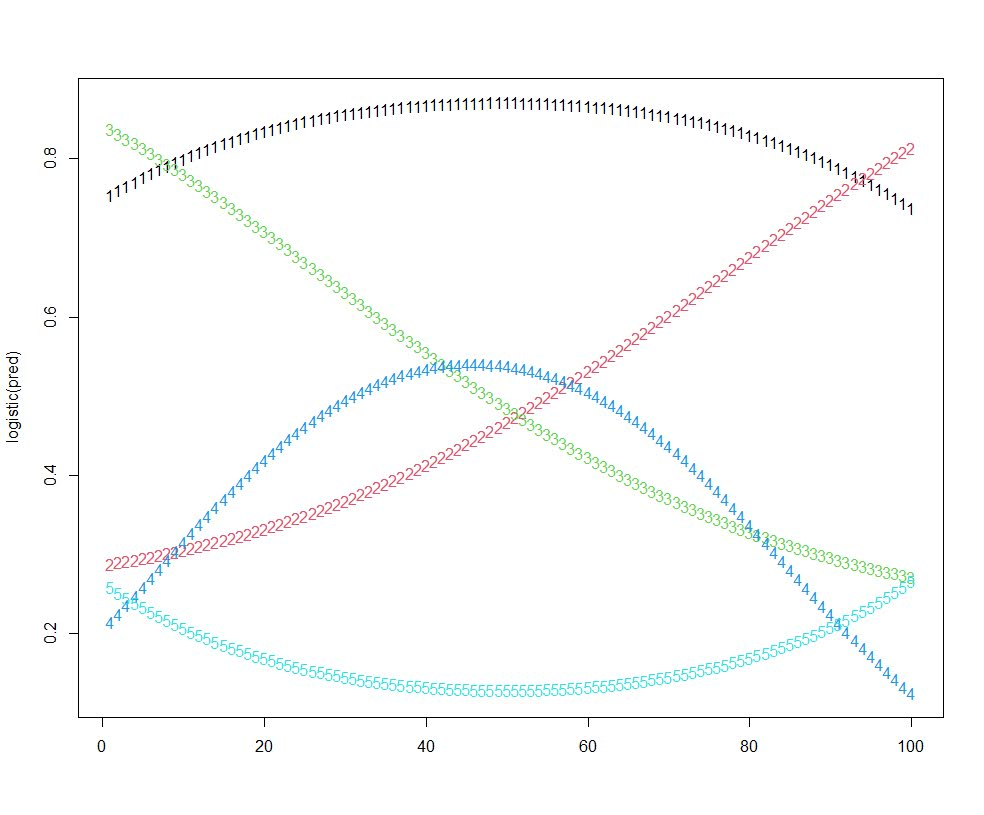These are quite similar to the curves for the paper I am reviewing, a consistent low probability (5), and a consistent high (1), a downward mostly linear slope (3), and an upward linear slope (2), and then one parabola concave down (4) (in the paper they had one concave up).

I figured the initial probability I assigned would highly impact the curve the model assigned a person to in this simulation. It ends up being more spread out than I expected though.

``````# distribution of classes vs original probability
ld\$clus <- clusters(mod)
r1 <- ld[ld\$time == 1,]
clustjit <- r1\$clus + runif(n,-0.2,0.2)
plot(clustjit,r1\$op) # more spread out than I thought``````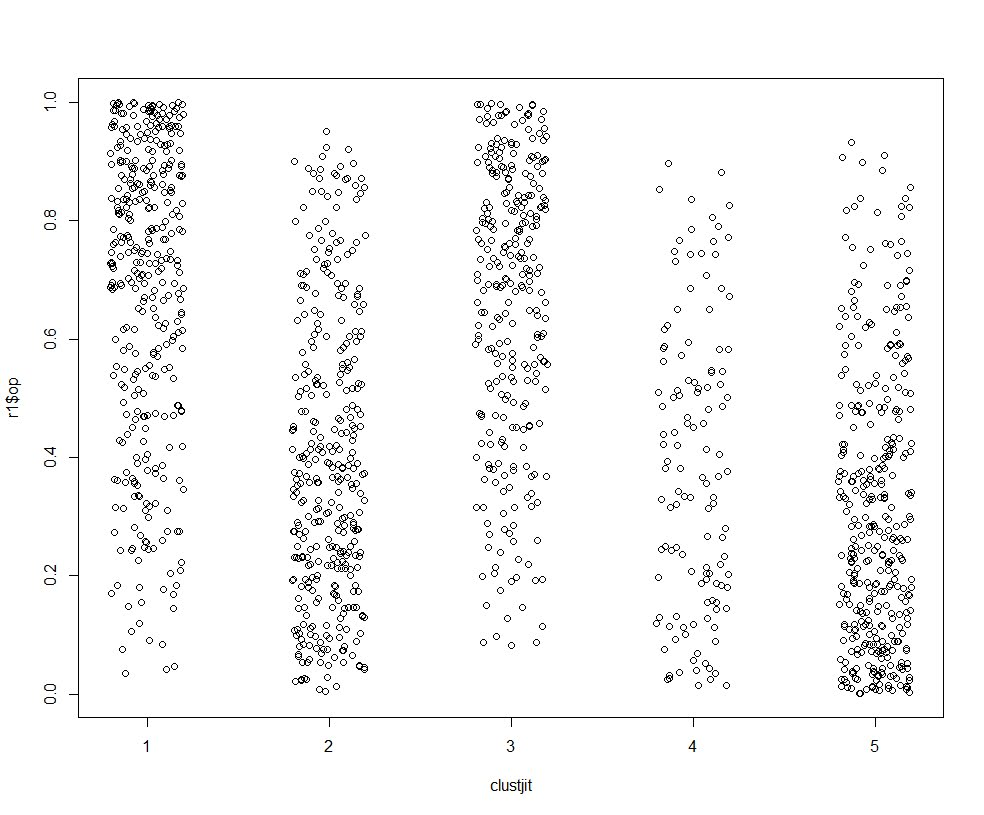So there is some tendency for each trajectory to be correlated based on the original probability, but it isn’t that strong.

If we look at the average max posterior probabilities, they are OK minus the parabola group 4.

``````# average posterior probability
pp <- data.frame(posterior(mod))
ld\$pp <- pp[cbind(1:(n*tp),ld\$clus)]
r1 <- ld[ld\$time == 1,]
aggregate(pp ~ clus, data = r1, mean)
#   clus        pp
# 1    1 0.8923801
# 2    2 0.7903938
# 3    3 0.7535281
# 4    4 0.6380946
# 5    5 0.8419221``````

The paper I am reviewing has much higher APPs for each group, so maybe they are really representing pop heterogeneity instead of continuous state dependence, it is just really hard with such observational data to tell the difference.

# Simulating Group Based Trajectories (in R)

The other day I pointed out on Erwin Kalvelagen’s blog how mixture models are a solution to fit regression models with multiple lines (where identification of which particular function/line is not known in advance).

I am a big fan of simulating data when testing out different algorithms for simply the reason it is often difficult to know how an estimator will behave with your particular data. So we have a bunch of circumstances with mixture models (in particular here I am focusing on repeated measures group based traj type mixture models) that it is hard to know upfront how they will do. Do you want to estimate group based trajectories, but have big N and small T? Or the other way, small N and big T? (Larger sample sizes tend to result in identifying more mixtures as you might imagine (Erosheva et al., 2014).) Do you have sparse Poisson data? Or high count Poisson data? Do you have 100,000 data points, and want to know how big of data and how long it may take? These are all good things to do a simulation to see how it behaves when you know the correct answer.

These are relevant no matter what the particular algorithm – so the points are all the same for k-medoids for example (Adepeju et al., 2021; Curman et al., 2015). Or whatever clustering algorithm you want to use in this circumstance. So here I show a few different simulations showing:

• GBTM can recover the correct underlying equations
• AIC/BIC fit stats have a difficult time distinguishing the correct number of groups
• If the underlying model is a random effects instead of latent clusters, AIC/BIC performs quite well

The last part is because GBTM models have a habit of spitting out solutions, even if the true underlying data process has no discrete groups. This is what Skardhamar (2010) did in his article. It was focused on life course, but it applies equally to the spatial analysis GBTM myself and others have done as well (Curman et al., 2015; Weisburd et al., 2004; Wheeler et al., 2016). I’ve pointed out in the past that even if the fit for GBTM looks good, the underlying data can suggest a random effects model will work quite well, and Greenberg (2016) makes pretty much the same point as well.

# Example in R

In the past I have shown how to use the crimCV package to fit these group based traj models, specifically zero-inflated Poisson models (Nielsen et al., 2014). Here I will show a different package, the R `flexmix` package (Grün & Leisch, 2007). This will be Poisson mixtures, but they have an example of doing zip models in there docs if you want.

So first, I load in the `flexmix` library, set the seed, and generate longitudinal data for three different Poisson models. One thing to note here, mixture models don’t assign an observation 100% to an underlying mixture, but the data I simulate here is 100% in a particular group.

``````################################################
library("flexmix")
set.seed(10)

# Generate simulated data
n <- 200 #number of individuals
t <- 10   #number of time periods
dat <- expand.grid(t=1:t,id=1:n)

# Setting up underlying 3 models
time <- dat\$t
p1 <- 3.5 - time
p2 <- 1.3 + -1*time + 0.1*time^2
p3 <- 0.15*time
p_mods <- data.frame(p1,p2,p3)

# Selecting one of these by random
# But have different underlying probs
latent <- sample(1:3, n, replace=TRUE, prob=c(0.35,0.5,0.15))
dat\$lat <- expand.grid(t=1:t,lat=latent)\$lat
dat\$sel_mu <- p_mods[cbind(1:(n*t), dat\$lat)]
dat\$obs_pois <- rpois(n=n*t,lambda=exp(dat\$sel_mu))
################################################``````

Now that is the hard part really – figuring out exactly how you want to simulate your data. Here it would be relatively simple to increase the number of people/areas or time period. It would be more difficult to figure out underlying polynomial functions of time.

Next part we fit a 3 mixture model, then assign the highest posterior probabilities back into the original dataset, and then see how we do.

``````################################################
# Now fitting flexmix model
mod3 <- flexmix(obs_pois ~ time + I(time^2) | id,
model = FLXMRglm(family = "poisson"),
data = dat, k = 3)
dat\$mix3 <- clusters(mod3)

# Seeing if they overlap with true labels
table(dat\$lat, dat\$mix3)/t
################################################``````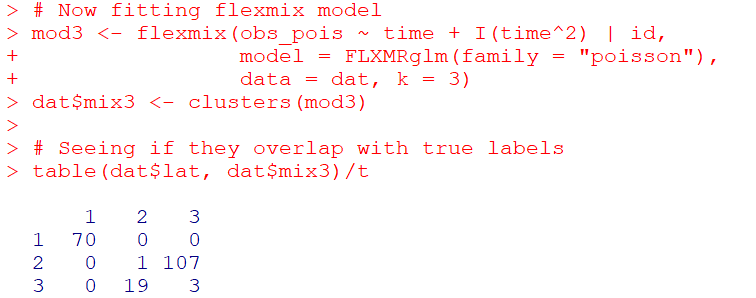So you can see that the identified groupings are quite good. Only 4 groups out of 200 are mis-placed in this example.

Next we can see if the underlying equations were properly recovered (you can have good separation between groups, but the polynomial fit may be garbage).

``````# Seeing if the estimated functions are close
rm3 <- refit(mod3)
summary(rm3)``````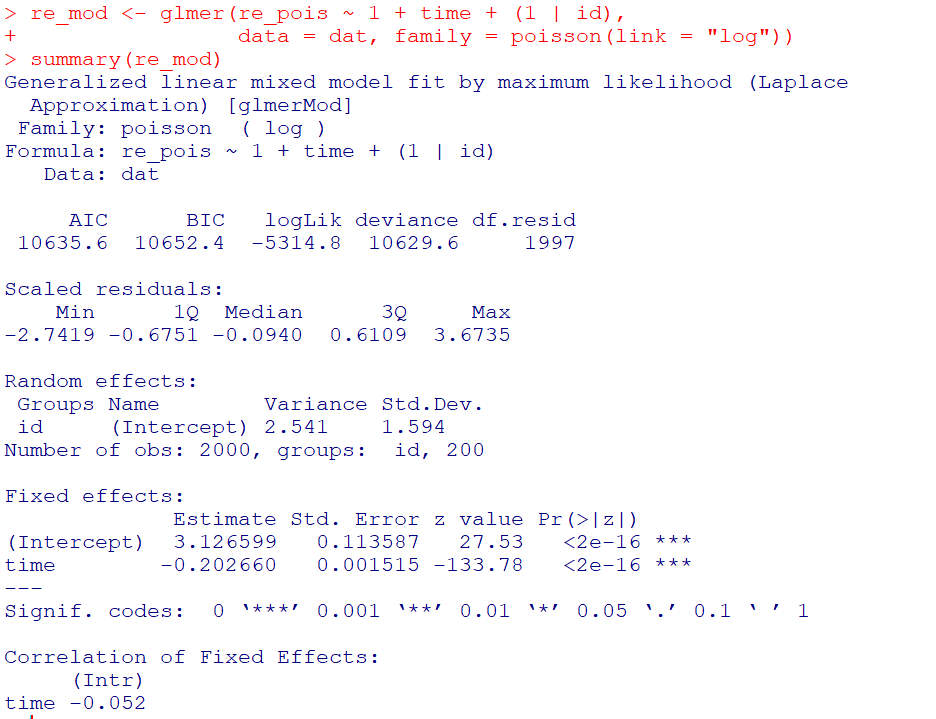This shows the equations are really as good as you could expect. The standard errors are as wide as they are because this isn’t really all that large a data sample for generalized linear models.

So this shows that if I feed in the correct underlying equation (almost, I could technically submit different equations with/without quadratic terms for example). But what about the real world situation in which you do not know the correct number of groups? Here I fit models for 1 to 8 groups, and then use the typical AIC/BIC to see which group it selects:

``````################################################
# If I look at different groups will AIC/BIC
# pick the right one?

group <- 1:8
left_over <- group[!(group %in% 3)]
aic <- rep(-1, 8)
bic <- rep(-1, 8)
aic <- AIC(mod3)
bic <- BIC(mod3)

for (i in left_over){
mod <- flexmix(obs_pois ~ time + I(time^2) | id,
model = FLXMRglm(family = "poisson"),
data = dat, k = i)
aic[i] <- AIC(mod)
bic[i] <- BIC(mod)
}

fit_stats <- data.frame(group,aic,bic)
fit_stats
################################################``````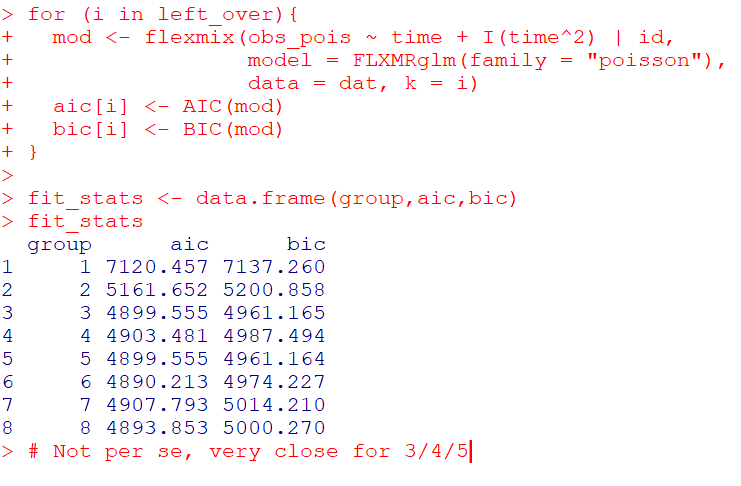Here it actually fit the same model for 3/5 groups (sometimes even if you tell flexmix to fit 5 groups, it will only return a smaller number). You can see that the fit stats for group 4 through are almost the same. So while AIC/BIC did technically pick the right number in this simulated example, it is cutting the margin pretty close to picking 4 groups in this data instead of 3.

So the simulation Skardhamar (2010) did was slightly different than this so far. What he did was simulate data with no underlying trajectory groups, and then showed GBTM tended to spit out solutions. Here I will show that is the case as well. I simulate random intercepts and a simple linear trend over time.

``````################################################
# Simulate random effects model
library(lme4)
rand_eff <- rnorm(n=n,0,1.5)
dat\$re <- expand.grid(t=1:t,re=rand_eff)\$re
dat\$re_pois <- rpois(n=n*t,lambda=exp(dat\$sel_mu))
dat\$mu_re <- 3 + -0.2*time + dat\$re
dat\$re_pois <- rpois(n=n*t,lambda=exp(dat\$mu_re))

re_mod <- glmer(re_pois ~ 1 + time + (1 | id),
data = dat, family = poisson(link = "log"))
summary(re_mod)
################################################``````So you can see that the random effects model is all fine and dandy – recovers both the fixed coefficients, as well as estimates the correct variance for the random intercepts.

So here I go and see how the AIC/BIC compares for the random effects models vs GBTM models for 1 to 8 groups (I stuff the random effects model in the first row for group 0):

``````################################################
# Test AIC/BIC for random effects vs GBTM
group <- 0:8
left_over <- 1:8
aic <- rep(-1, 9)
bic <- rep(-1, 9)
aic <- AIC(re_mod)
bic <- BIC(re_mod)

for (i in left_over){
mod <- flexmix(re_pois ~ time + I(time^2) | id,
model = FLXMRglm(family = "poisson"),
data = dat, k = i)
aic[i+1] <- AIC(mod)
bic[i+1] <- BIC(mod)
}

fit_stats <- data.frame(group,aic,bic)
fit_stats
################################################``````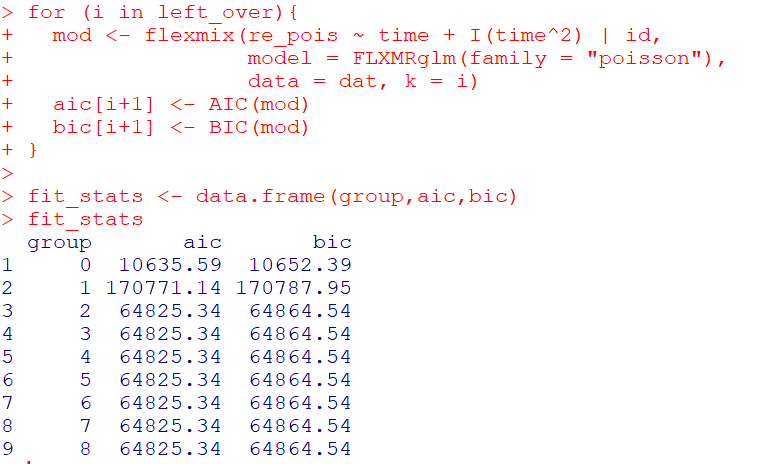So it ends up flexmix will not give us any more solutions than 2 groups. But that the random effect fit is so much smaller (either by AIC/BIC) than the GBTM you wouldn’t likely make that mistake here.

I am not 100% sure how well we can rely on AIC/BIC for these different models (R does not count the individual intercepts as a degree of freedom here, so k=3 instead of k=203). But no reasonable accounting of k would flip the AIC/BIC results for these particular simulations.

One of the things I will need to experiment with more, I really like the idea of using out of sample data to validate these models instead of AIC/BIC – no different than how Nielsen et al. (2014) use leave one out CV. I am not 100% sure if that is possible in this set up with `flexmix`, will need to investigate more. (You can have different types of cross validation in that context, leave entire groups out, or forecast missing data within an observed group.)

Adepeju, M., Langton, S., & Bannister, J. (2021). Anchored k-medoids: a novel adaptation of k-medoids further refined to measure long-term instability in the exposure to crime. Journal of Computational Social Science, 1-26.

Grün, B., & Leisch, F. (2007). Fitting finite mixtures of generalized linear regressions in R. Computational Statistics & Data Analysis, 51(11), 5247-5252.

Curman, A. S., Andresen, M. A., & Brantingham, P. J. (2015). Crime and place: A longitudinal examination of street segment patterns in Vancouver, BC. Journal of Quantitative Criminology, 31(1), 127-147.

Erosheva, E. A., Matsueda, R. L., & Telesca, D. (2014). Breaking bad: Two decades of life-course data analysis in criminology, developmental psychology, and beyond. Annual Review of Statistics and Its Application, 1, 301-332.

Greenberg, D. F. (2016). Criminal careers: Discrete or continuous?. Journal of Developmental and Life-Course Criminology, 2(1), 5-44.

Nielsen, J. D., Rosenthal, J. S., Sun, Y., Day, D. M., Bevc, I., & Duchesne, T. (2014). Group-based criminal trajectory analysis using cross-validation criteria. Communications in Statistics-Theory and Methods, 43(20), 4337-4356.

Skardhamar, T. (2010). Distinguishing facts and artifacts in group-based modeling. Criminology, 48(1), 295-320.

Weisburd, D., Bushway, S., Lum, C., & Yang, S. M. (2004). Trajectories of crime at places: A longitudinal study of street segments in the city of Seattle. Criminology, 42(2), 283-322.

Wheeler, A. P., Worden, R. E., & McLean, S. J. (2016). Replicating group-based trajectory models of crime at micro-places in Albany, NY. Journal of Quantitative Criminology, 32(4), 589-612.

# New book: Micro geographic analysis of Chicago homicides, 1965-2017

In joint work with Chris Herrmann and Dick Block, we now have a book out – Understanding Micro-Place Homicide Patterns in Chicago (1965 – 2017). It is a Springer Brief book, so I recommend anyone who has a journal article that is too long that this is a potential venue for the work. (Really this is like the length of three journal articles.)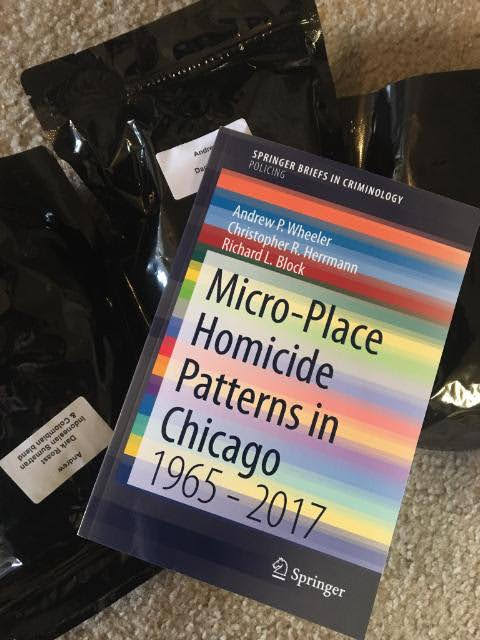A few things occurred to prompt me to look into this. First, Chicago increased a big spike of homicides in 2016 and 2017. Here is a graph breaking them down between domestic related homicides and all other homicides. You can see all of the volatility is related to non-domestic homicides.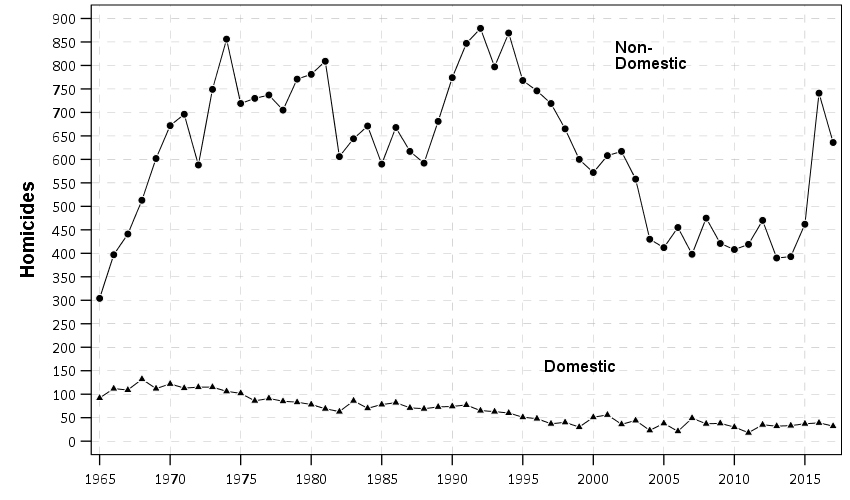So this (at least to me) begs the question of whether those spiked homicides show similar characteristics compared to historical homicides. Here we focus on long term spatial patterns and micro place grid cells in the city, 150 by 150 meter cells. Dick & Carolyn Block had collated data, including the address where the body was discovered, using detective case notes starting in 1965 (ending in 2000). The data from 2000 through 2017 is the public incident report data released by Chicago PD online. Although Dick and Carolyn’s public dataset is likely well known at this point, Dick has more detailed data than is released publicly on ICPSR and a few more years (through 2000). Here is a map showing those homicide patterns aggregated over the entire long time period.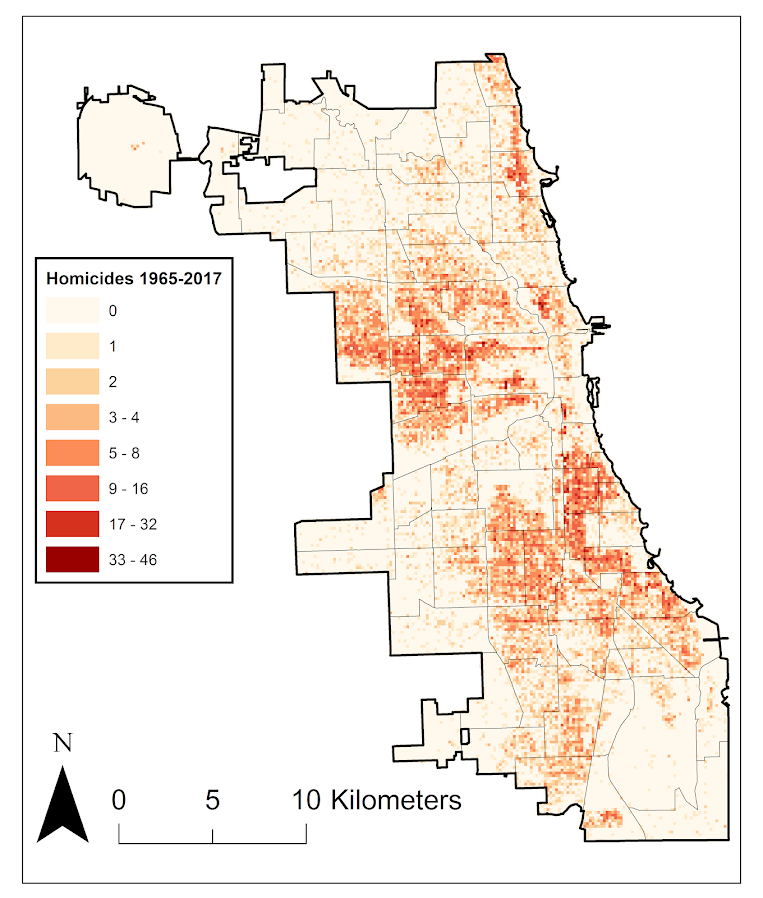So we really have two different broad exploratory analyses we employed in the work. One was to examine homicide clustering, and the other was to examine temporal patterns in homicides. For clustering, we go through a ton of different metrics common in the field, and I introduce even one more, Theil’s decomposition for within/between neighborhood clustering. This shows Theil’s clustering metric within neighborhoods in Chicago (based on the entire time period).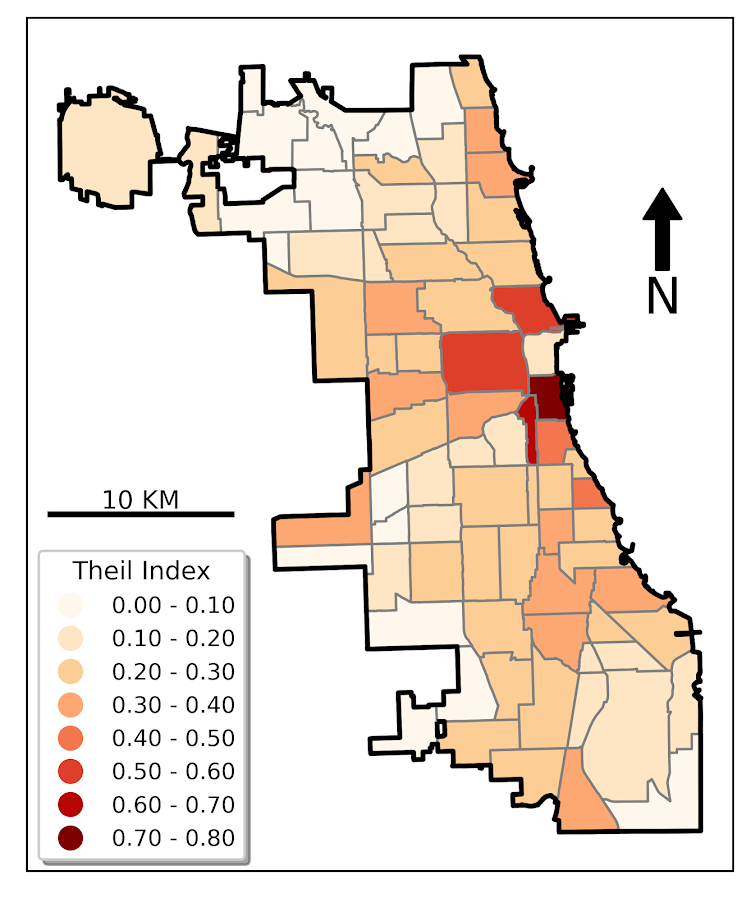So areas around the loop showed more clustering in homicides, but here it appears it is somewhat confounded with neighborhood size – smaller neighborhoods appear to have more clustering. This is sort of par for the course for these clustering metrics (we go through several different Gini variants as well), in that they are pretty fickle. You do a different temporal slice of data or treat empty grid cells differently the clustering metrics can change quite a bit.

So I personally prefer to focus on long term temporal patterns. Here I estimated group based trajectory models using zero-inflated Poisson models. And here are the predicted outputs for those grid cells over the city. You can see unlike prior work David Weisburd (Seattle), myself (Albany), or Martin Andresen (Vancouver) has done, they are much more wavy patterns. This may be due to looking over a much longer horizon than any of those prior works though have.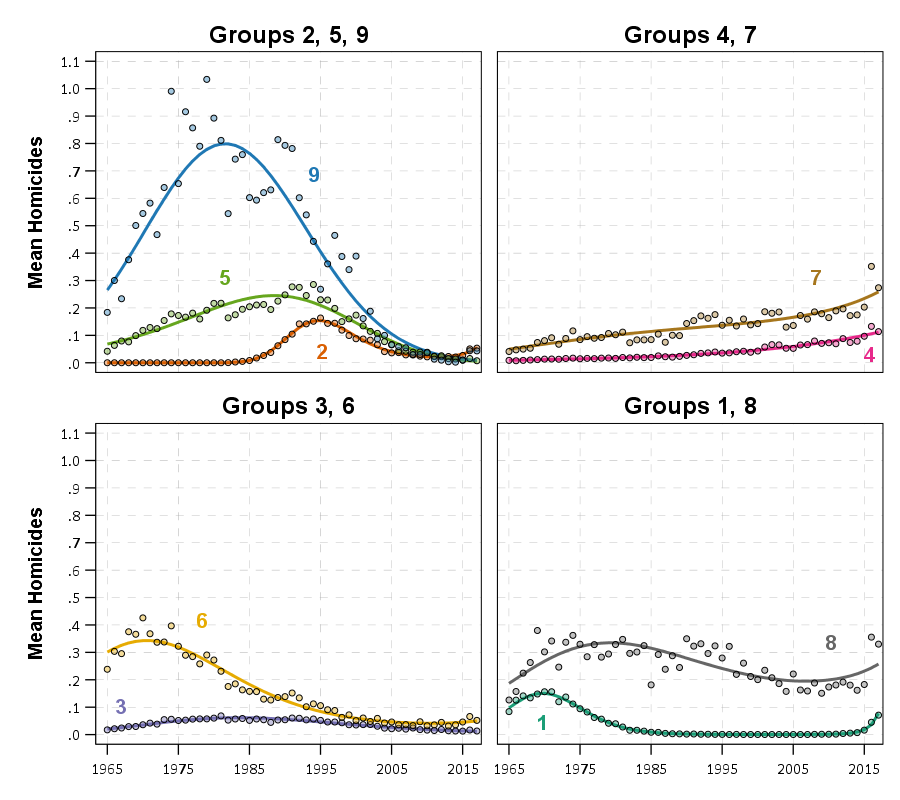The big wave, Group 9, ends up being clearly tied to former large public housing projects, which their demolitions corresponds to the downturn.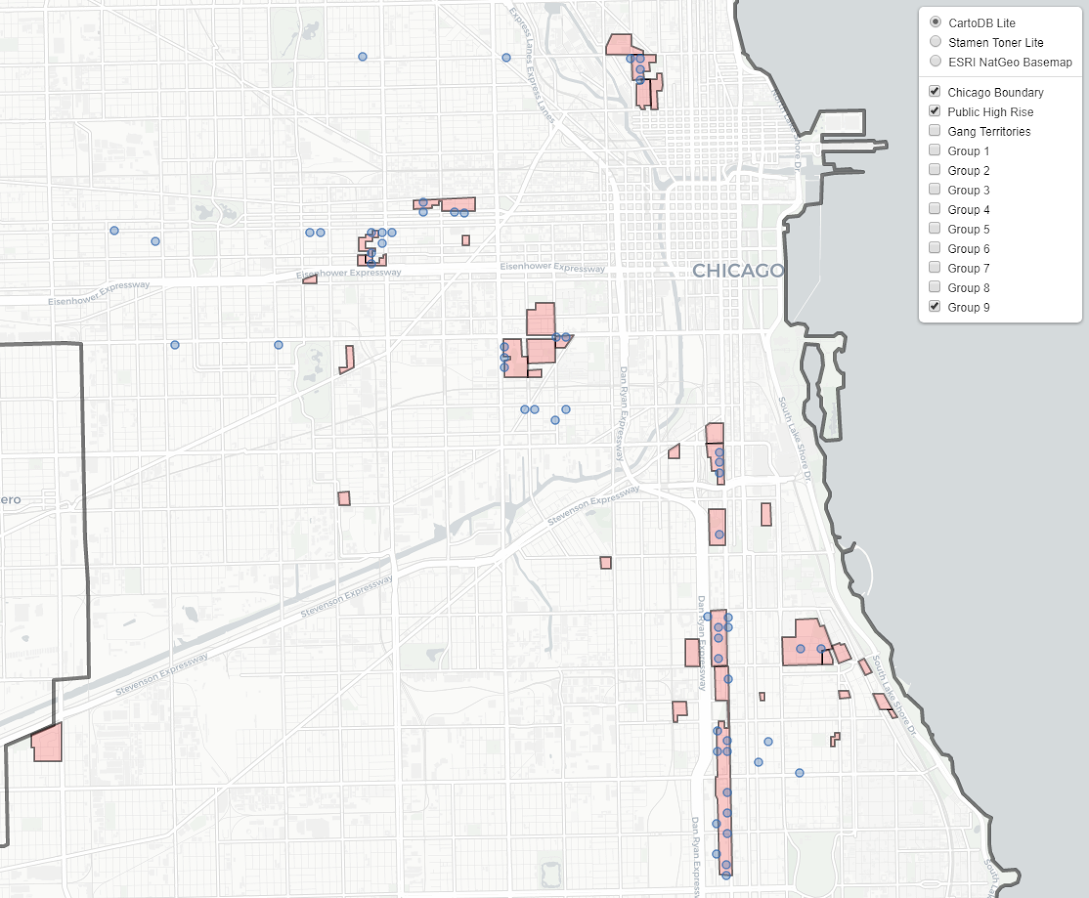I have an interactive map to explore the other trajectory groups here. Unfortunately the others don’t show as clear of patterns as Group 9, so it is difficult to answer any hard questions about the uptick in 2016/2017, you could find evidence of homicides dispersing vs homicides being in the same places but at a higher intensity if you slice the data different ways.

Unfortunately the analysis is never ending. Chicago homicides have again spiked this year, so maybe we will need to redo some analysis to see if the more current trends still hold. I think I will migrate away from the clustering metrics though (Gini and Theil), they appear to be too volatile to say much of anything over short term patterns. I think there may be other point pattern analysis that are more diagnostic to really understand emerging/changing spatial patterns.

The coffee next to the cover image is Chris Herrmann’s beans, so go get yourself some as well at Fellowship Coffee!

# Using pytorch to estimate group based traj models

Deep learning, tensors, pytorch. Now that I have that seo junk out of the way 🙂 – I’ve been trying to teach myself some “Deep Learning”, as it is what all of the cool kids are doing these days.

I was having a hard time though with many of the different examples. Many are for image data, and so it was hard for me to translate that to actual applications I am interested in. Many do talk about dimension reduction and reducing to hidden layers, so I thought that was similar in nature to latent class analysis, such as group-based-trajectory-modelling (GBTM).

If you aren’t familiar with GBTM, imagine a scenario in which you cluster data, and then you estimate a different regression model to predict some outcome for each subset of the clustered data. This is just a way to do that whole set-up in one go, instead of doing each part separately. It has quite a few different names – latent class analysis and mixture modelling are two common ones. The only thing really different about GBTM is that you have repeated observations – so if you follow the same person over time, they should always be assigned to the same cluster/mixture.

In short you totally can do GBTM models in deep learning libraries (as I will show), but actually most examples that I have walked through are more akin to dimension reduction of columns (so like PCA/Canonical Correlation). But the deep learning libraries are flexible enough to do the latent class analysis I want here. As far as I can tell they are basically just a nice way to estimate systems of equations (with a ton of potential parameters, and do it on the GPU if you want).

So I took it as a challenge to estimate GBTM models in a deep learning library – here pytorch. In terms of the different architectures/libraries (e.g. pytorch, tensorflow, Vowpal Wabbit) I just chose pytorch because one of my co-workers suggested pytorch was easier to learn!

I’ve posted a more detailed notebook of the code, but it worked out quite well. So first I simulated two groups of data (50 observations in each group and 11 time periods). I added a tiny bit of random noise, so this (I was hoping) should be a pretty tame problem for the machine to learn.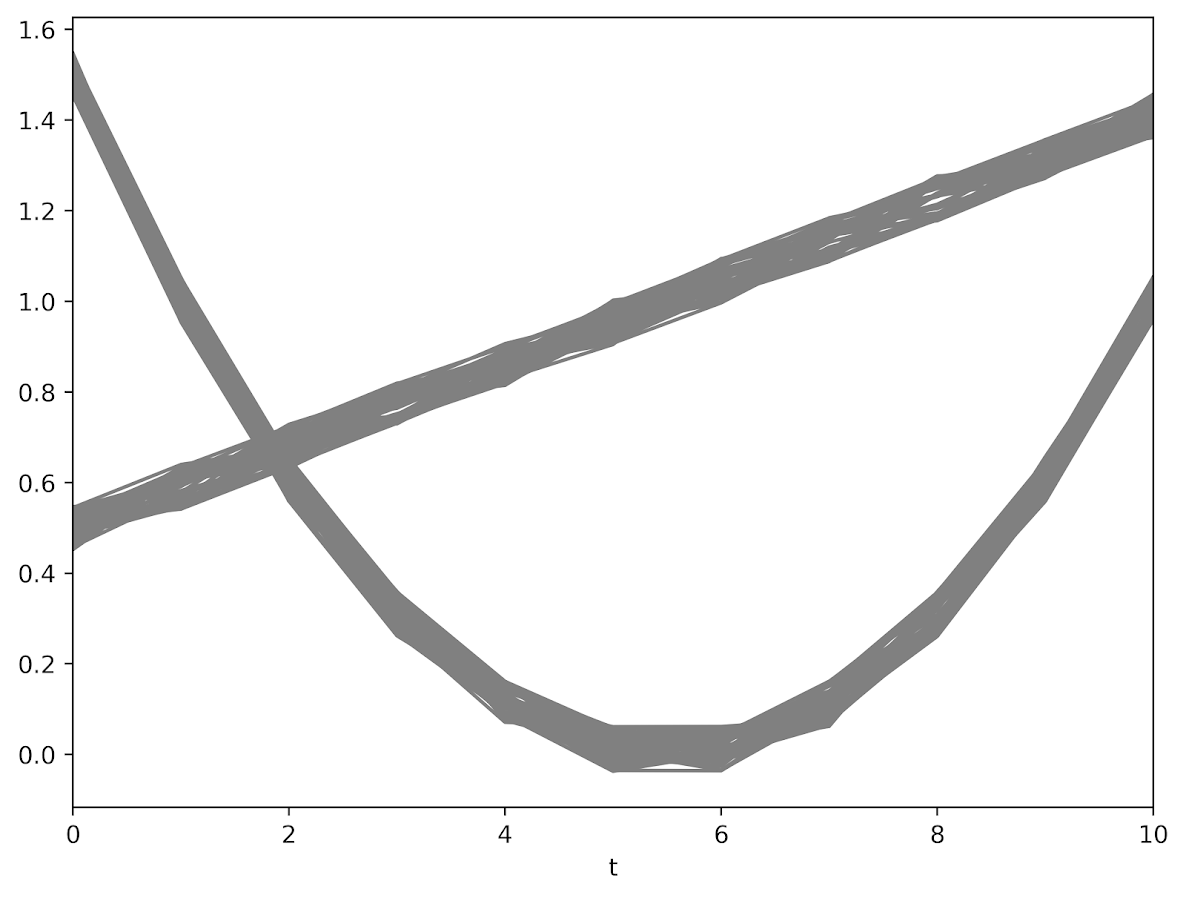The code to generate a pytorch module and have the machine churn out the gradients is pretty slick (less than 30 lines total of non-comments). Many GBTM code bases make you do the analysis in wide format (so one row is an observation), but here I was able to figure out how to set it up in long data format, which makes it real easy to generalize to unbalanced data.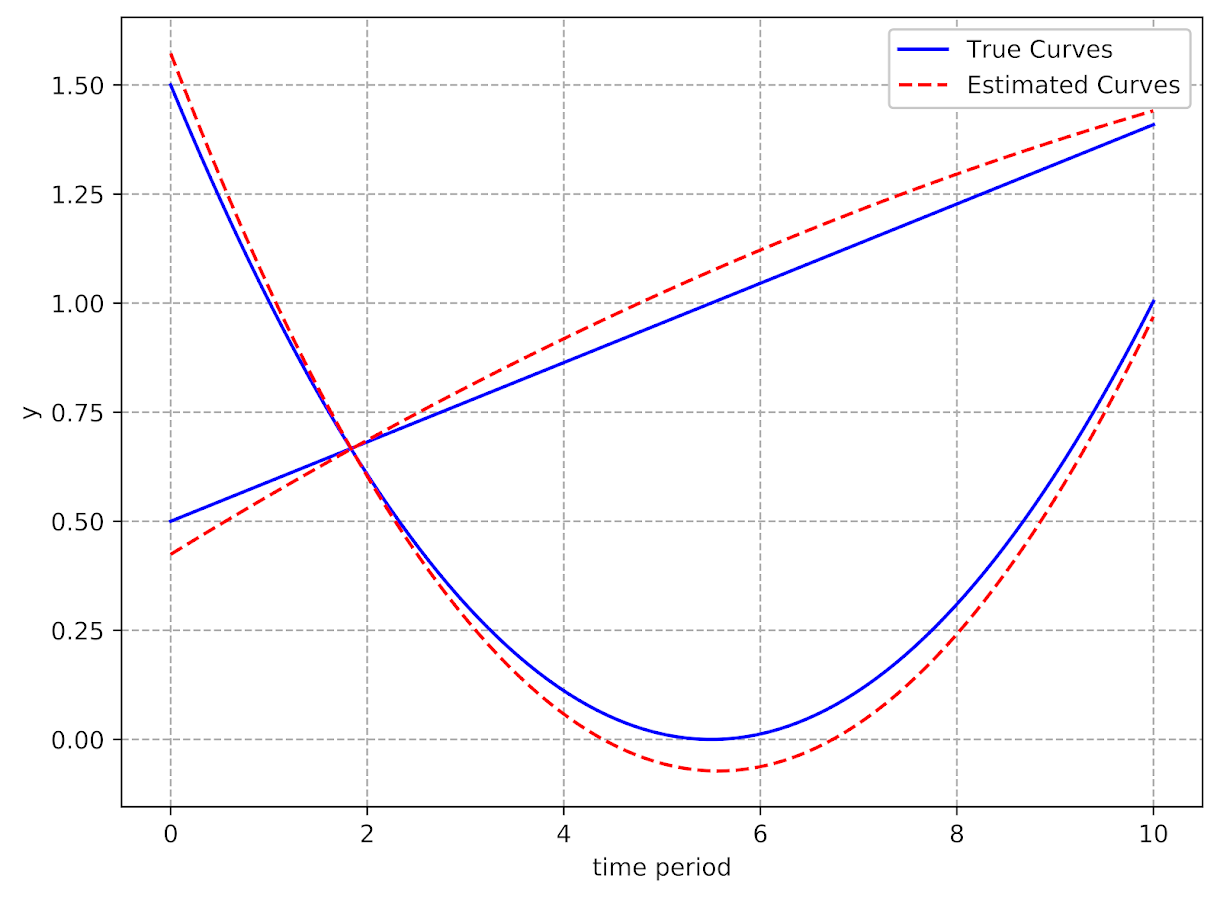It took quite a few iterations to converge though, (iterations were super fast, but it is a tiny problem, so not sure how timing will generalize) and only converged when using the Adam optimizer (stochastic gradient descent converged to an answer with a similar mean square error, but not to anywhere near the right answer). These models are notorious for converging to sub-optimal locations, so that may just be an intrinsic part of the problem and a good library needs to do better with starting conditions.

I have a few notes about potential updates to the code at the end of my Jupyter notebook. For count or binomial 0/1 data, that should be a pretty easy update. Also need to write code to do out of sample predictions (which I think I can figure out as well). A harder problem I am not sure how to figure out is to do an equation for the latent groups inside of the function. And I don’t know how to get standard errors for the coefficient estimates. Hopefully I can figure that out while trying to teach myself some more deep learning. I have a few convolution ideas I want to try out for spatial-temporal crime forecasting and include proactive police feedback, but I won’t get around to them for quite awhile I imagine.

# Some notes on PChange – estimating when trajectories cross over time

J.C. Barnes and company published a paper in JQC not too long ago and came up with a metric, PChange, to establish the number of times trajectories cross in a sample. This is more of interest to life course folks, although it is not totally far fetched to see it applied to trajectories of crime at places. Part of my interest in it was simply that it is an interesting statistical question — when two trajectories with errors cross. A seemingly simple question that has a few twists and turns. Here are my subsequent notes on that metric.

# The Domain Matters

First, here is an example of the trajectories not crossing: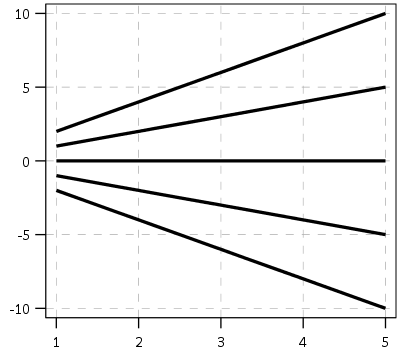This points to an important assumption about those lines not crossing though that was never mentioned in the Barnes paper — the domain matters. For instance, if we draw those rays further back in time what happens?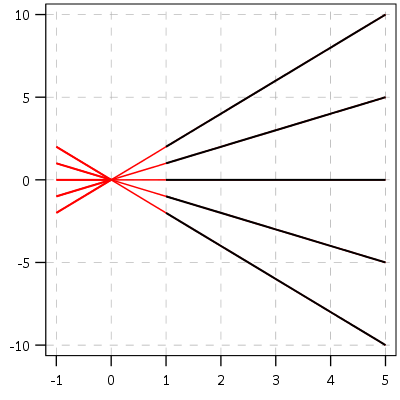They cross! This points to an important piece of information when evaluating PChange — the temporal domain in which you examine the data matters. So if you have a sample of juvenile delinquency measures from 14-18 you would find less change than a similar sample from 12-20.

This isn’t really a critique of PChange — it is totally reasonable to only want to examine changes within a specific domain. Who cares if delinquency trajectories cross when people are babies! But it should be an important piece of information researchers use in the future if they use PChange — longer samples will show more change. It also won’t be fair to compare PChange for samples of different lengths.

# A Functional Approach to PChange

For above you may ask — how would you tell if a trajectory crosses outside of the domain of the data? The answer to that question is you estimate an underlying function of the trajectory — some type of model where the outcome is a function of age (or time). With that function you can estimate the trajectory going back in time or forward in time (or in between sampled measurements). You may not want to rely on data outside of the domain (its standard error will be much higher than data within the time domain, forecasting is always fraught with peril!), but the domain of your sample is ultimately arbitrary. So what about the question will the trajectories ever cross? Or would the trajectories have crossed if I had data for ages 12-20 instead of just 16-18? Or would they have crossed if I checked the juveniles at age 16 1/2 instead of only at 16?

So actually instead of the original way the Barnes paper formulated PChange, here is how I thought about calculating PChange. First you estimate the underlying trajectory for each individual in your sample, then you take the difference of those trajectories.

``````y_i = f(t)
y_j = g(t)
y_delta = f(t) - g(t) = d(t)``````

Where `y_i` is the outcome `y` for observation `i`, and `y_j` is the outcome `y` for observation `j`. `t` is a measure of time, and thus the anonymous functions `f` and `g` represent growth models for observations i and j over time. `y_delta` is then the difference between these two functions, which I represent as the new function `d(t)`. So for example the functions for each individual might be quadratic in time:

``````y_i = b0i + b1i(t) + b2i(t^2)
y_j = b0j + b1j(t) + b2j(t^2)``````

Subsequently the difference function will also be quadratic, and can be simply represented as:

``y_delta = (b0i - b0j) + (b1i - b1j)*t + (b2i - b2j)*t^2``

Then for the trajectories to cross (or at least touch), `y_delta` just then has to equal zero at some point along the function. If this were math, and the trajectories had no errors, you would just set d(t) = 0 and solve for the roots of the equation. (Most people estimating models like these use functions that do have roots, like polynomials or splines). If you cared about setting the domain, you would then just check if the roots are within the domain of interest — if they are, the trajectories cross, if they are not, then they do not cross. For data on humans with age, obviously roots for negative human years will not be of interest. But that is a simple way to solve the domain problem – if you have an underlying estimate of the trajectory, just see how often the trajectories cross within equivalent temporal domains in different samples.

I’d note that the idea of having some estimate of the underlying trajectory is still relevant even within the domain of the data — not just extrapolating to time periods outside. Consider two simple curves below, where the points represent the time points where each individual was measured.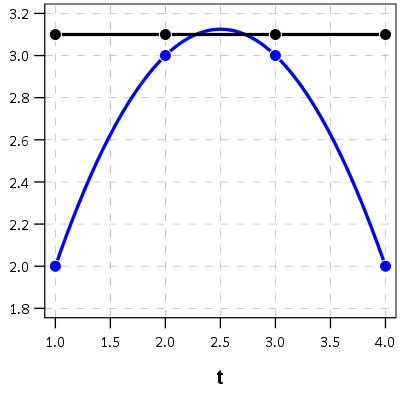So while the two functions cross, when only considering the sampled locations, like is done in Barnes et al.’s PChange, you would say these trajectories do not cross, when in actuality they do. It is just the sampled locations are not at the critical point in the example for these two trajectories.

This points to another piece of contextual information important to interpreting PChange — the number of sample points matter. If you have samples every 6 months, you will likely find more changes than if you just had samples every year.

I don’t mean here to bag on Barnes original metric too much — his PChange metric does not rely on estimating the underlying functional form, and so is a non-parametric approach to identifying change. But estimating the functional form for each individual has some additional niceties — one is that you do not need the measures to be at equivalent sample locations. You can compare someone measured at 11, 13, and 18 to someone who is measured at 12, 16, and 19. For people analyzing stuff for really young kids I bet this is a major point — the underlying function at a specific age is more important then when you conveniently measured the outcome. For older kids though I imagine just comparing the 12 to 11 year old (but in the same class) is probably not a big deal for delinquency. It does make it easier though to compare say different cohorts in which the measures are not at nice regular intervals (e.g. Add Health, NLYS, or anytime you have missing observations in a longitudinal survey).

In the end you would only want to estimate an underlying functional form if you have many measures (more so than 3 in my example), but this typically ties in nicely with what people modeling the behavior over time are already doing — modeling the growth trajectories using some type of functional form, whether it is a random effects model or a group based trajectory etc., they give you an underlying functional form. If you are willing to assume that model is good enough to model the trajectories over time, you should think it is good enough to calculate PChange!

# The Null Matters

So this so far would be fine and dandy if we had perfect estimates of the underlying trajectories. We don’t though, so you may ask, even though `y_delta` does not exactly equal zero anywhere, its error bars might be quite wide. Wouldn’t we then still infer that there is a high probability the two trajectories cross? This points to another hidden assumption of Barnes PChange — the null matters. In the original PChange the null is that the two trajectories do not cross — you need a sufficient change in consecutive time periods relative to the standard error to conclude they cross. If the standard error is high, you won’t consider the lines to cross. Consider the simple table below:

``````Period A_Level A_SE B_Level B_SE
1      4         1    1.5   0.5
2      5         1    3     0.5
3      6         1    4.5   0.5
4      7         1    6     0.5``````

Where A_Level and B_Level refer to the outcome for the four time periods, and A_SE and B_SE refer to the standard errors of those measurements. Here is the graph of those two trajectories, with the standard error drawn as areas for the two functions (only plus minus one standard error for each line).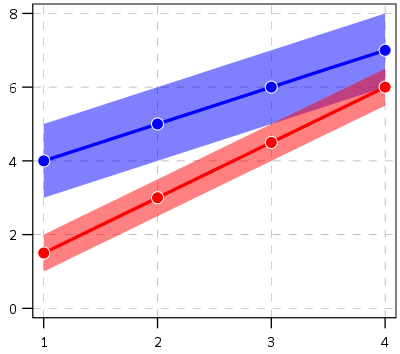And here is the graph of the differences — assuming that the covariance between the two functions is zero (so the standard error of the difference equals `sqrt(A_SE^2 + B_SE^2)`). Again only plus/minus one standard error.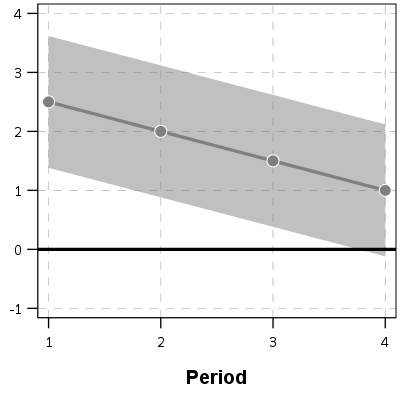You can see that the line never crosses zero, but the standard error area does. If our null is `H0: y_delta = 0 for any t`, then we would fail to reject the null in this example. So in Barnes original PChange these examples lines would not cross, whereas with my functional approach we don’t have enough data to know they don’t cross. This I suspect would make a big difference in many samples, as the standard error is going to be quite large unless you have very many observations and/or very many time points.

If one just wants a measure of crossed or did not cross, with my functional approach you could set how wide you want to draw your error bars, and then estimate whether the high or low parts of that bar cross zero. You may not want a discrete measure though, but a probability. To get that you would integrate the probability over the domain of interest and calculate the chunk of the function that cross zero. (Just assume the temporal domain is uniform across time.)

So in 3d, my difference function would look like this, whereas to the bottom of the wall is the area to calculate the probability of the lines crossing, and the height of the surface plot is the PDF at that point. (Note the area of the density is not normalized to sum to 1 in this plot.)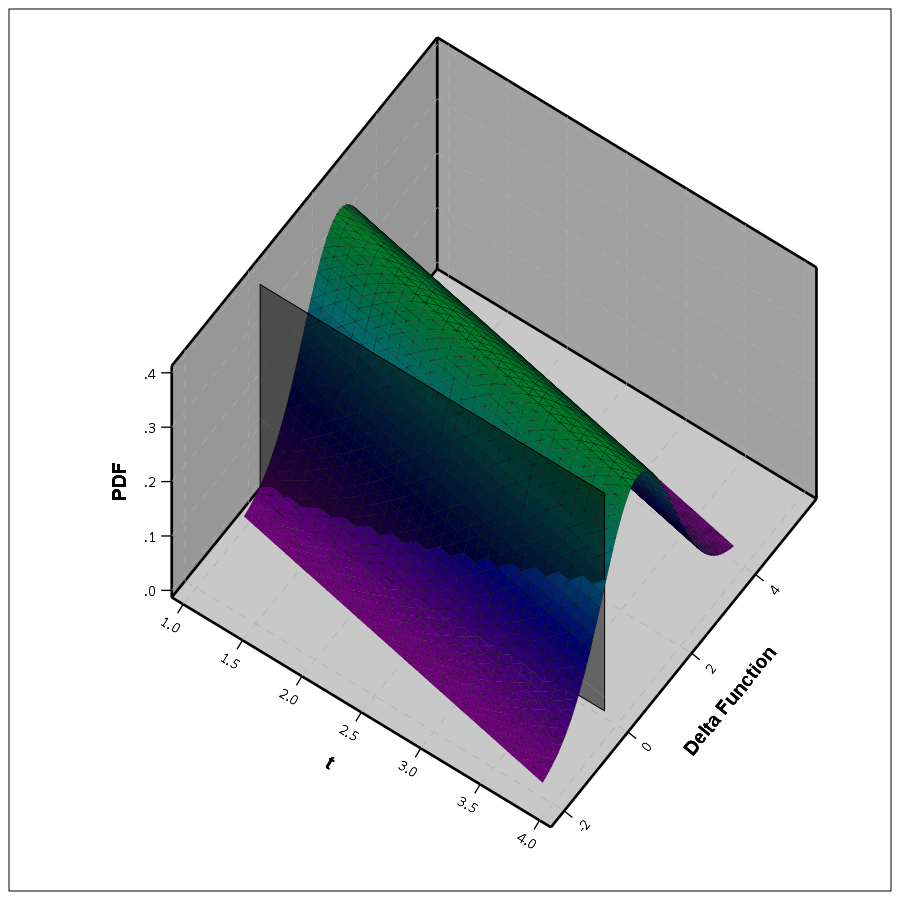This surface graph ends up crossing more than was observed in my prior 2d plots, as I was only plotting 1 standard error. Here imagine that the top green part of the density is the mean function — which does not cross zero — but then you have a non-trivial amount of the predicted density that does cross the zero line.

In the example where it just crosses one time by a little, it seems obvious to consider the small slice as the probability of the two lines crossing. I think to extend this to not knowing to test above or below the line you could calculate the probability on either side of the line, take the minimum, and then double that minimum for your p-value. So if say 5% of the area is below the line in my above example, you would double it and say the two-tailed p-value of the lines crossing is p = 0.10. Imagine the situation in which the line mostly hovers around 0, so the mass is about half on one side and half on the other. In that case the probability the lines cross seems much higher than 50%, so doubling seems intuitively reasonable.

So if you consider this probability to be a p-value, with a very small p-value you would reject the null that the lines cross. Unlike most reference distributions for p-values though, you can get a zero probability estimate of the lines crossing. You can aggregate up those probabilities as weights when calculating the overall PChange for the sample. So you may not know for certain if two trajectories cross, but you may be able to say these two trajectories cross with a 30% probability.

Again this isn’t to say that PChange is bad — it is just different. I can’t give any reasoning whether assuming they do cross (my functional approach) or assuming they don’t cross (Barnes PChange) is better – they are just different, but would likely make a large difference in the estimated number of crossings.

# Population Change vs Individual Level Change

So far I have just talked about trying to determine whether two individual lines cross. For my geographic analysis of trajectories in which I have the whole population (just a sample in time), this may be sufficient. You can calculate all pairwise differences and then calculate PChange (I see no data based reason to use the permutation sample approach Barnes suggested – we don’t have that big of samples, we can just calculate all pairwise combinations.)

But for many of the life course researchers, they are more likely to be interested in estimating the population of changes from the samples. Here I will show how you can do that for either random effects models, or for group based trajectory models based on the summary information. This takes PChange from a sample metric to a population level metric implied via your models you have estimated. This I imagine will be much easier to generalize across samples than the individual change metrics, which will be quite susceptible to outlier trajectories, especially in small samples.

First lets start with the random effects model. Imagine that you fit a linear growth model — say the random intercept has a variance of 2, and the random slope has a variance of 1. So these are population level metrics. The fixed effects and the covariance between the two random effect terms will be immaterial for this part, as I will discuss in a moment.

First, trivially, if you selected two random individuals from the population with this random effects distribution, the probability their underlying trajectories cross at some point is 1. The reason is for linear models, two lines only never cross if the slopes are perfectly parallel. Which sampling from a continuous random distribution has zero probability of them being exactly the same. This does not generalize to more complicated functions (imagine parabolas concave up and concave down that are shifted up and down so they never cross), but should be enough of a motivation to make the question only relevant for a specified domain of time.

So lets say that we are evaluating the trajectories over the range `t = [10,20]`. What is the probability two individuals randomly sampled from the population will cross? So again with my functional difference approach, we have

``````y_i = b0i + b1i*t
y_j = b0j + b1j*t
y_delta = (b0i - b0j) + (b1i - b1j)*t``````

Where in this case the `b0` and `b1` have prespecified distributions, so we know the distribution of the difference. Note that in the case with no covariates, the fixed effects will cancel out when taking the differences. (Generalizing to covariates is not as straightforward, you could either assume they are equal so they cancel out, or you could have them vary according to additional distributions, e.g. males have an 90% chance of being drawn versus females have a 10% chance, in that case the fixed effects would not cancel out.) Here I am just assuming they cancel out. Additionally, taking the difference in the trajectories also cancels out the covariance term, so you can assume the covariance between `(b0i - b0j)` and `(b1i - b1j)` is zero even if `b0` and `b1` have a non-zero covariance for the overall model. (Post is long enough — I leave that as an exercise for the reader.)

For each of the differences the means will be zero, and the variance will be the two variances added together, e.g. `b0i - b0j` will have a mean of zero and a variance of `2 + 2 = 4`. The variance of the difference in slopes will then be 2. Now to figure out when the two lines will cross.

If you make a graph where the X axis is the difference in the intercepts, and the Y axis is the difference in the slopes, you can then mark off areas that indicate the two lines will cross given the domain. Here for example is a sampling of where the lines cross – red is crossing, grey is not crossing.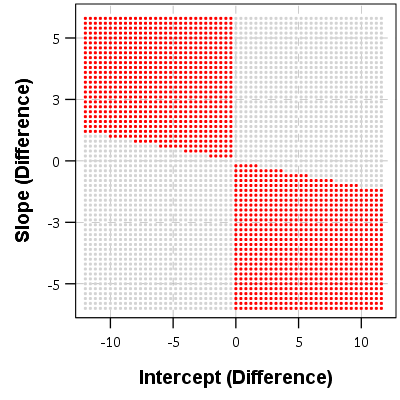So for example, say we had two random draws:

``````y_i = 1   + 0.5*t
y_j = 0.5 + 0.3*t
y_delta = 0.5 + 0.2*t``````

This then shows that the two lines do not cross when only evaluating `t` between 10 and 20. They have already diverged that far out (you would need negative `t` to have the lines cross). Imagine if `y_delta = -6 + 0.2*t` though, this line does cross zero though, at `t = 10` this function equals -1, whereas at `t = 20` the function equals 4.

If you do another 3d plot you can plot the bivariate PDF. Again integrate the chunks of areas in which the function crosses zero, and voila, you get your population estimate.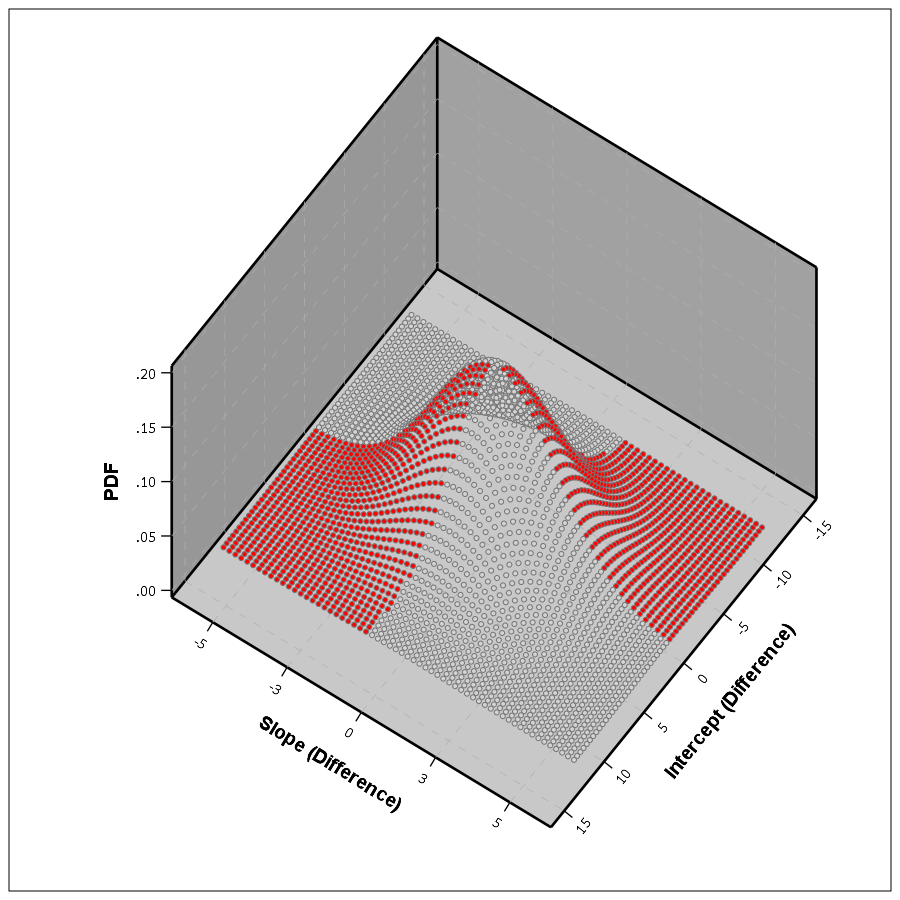This works in a similar manner to higher order polynomials, but you can’t draw it in a nice graph though. I’m blanking at the moment of a way to find these areas offhand in a nice way — suggestions welcome!

This gets a bit tricky thinking about in relation to individual level change. This approach does not assume any error in the random draws of the line, but assumes the draws will have a particular distribution. So the PChange does not come from adding up whether individual lines in your sample cross, it comes from the estimated distribution of what the difference in two randomly drawn lines would look like that is implied by your random effects model. Think if based on your random effect distribution you randomly drew two lines, calculated if they crossed, and then did this simulation a very large number of times. The integrations I’m suggesting are just an exact way to calculate PChange instead of the simulation approach.

If you were to do individual change from your random effects model you would incorporate the standard error of the estimated slope and intercept for the individual observation. This is for your hypothetical population though, so I see no need to incorporate any error.

Estimating population level change from group based trajectory models via my functional approach is more straightforward. First, with my functional approach you would assume individuals who share the same latent trajectory will cross with a high probability, no need to test that. Second, for testing whether two individual trajectories cross you would use the approach I’ve already discussed around individual lines and gain the p-value I mentioned.

So for example, say you had a probability of 25% that a randomly drawn person from group A would cross a randomly drawn person from Group B. Say also that Group A has 40/100 of the sample, and Group B is 60/100. So then we have three different groups: A to A, B to B, and A to B. You can then break down the pairwise number in each group, as well as the number of crosses below.

``````Compare   N    %Cross Cross
A-A      780    100    780
B-B     1770    100   1770
A-B     2400     25    600
Total   4950     64   3150``````

So then we have a population level p-change estimate of 64% from our GBTM. All of these calculations can be extended to non-integers, I just made them integers here to simplify the presentation.

Now, that overall PChange estimate may not be real meaningful for GBTM, as the denominator includes pairwise combinations of folks in the same trajectory group, which is not going to be of much interest. But just looking at the individual group solutions and seeing what is the probability they cross could be more informative. For example, although Barnes shows the GBTM models in the paper as not crossing, depending on how wide the standard errors of the functions are (that aren’t reported), this functional approach would probably assign non-zero probability of them crossing (think low standard error for the higher group crossing a high standard error for the low group).

Phew — that was a long post! Let me know in the comments if you have any thoughts/concerns on what I wrote. Simple question — whether two lines cross — not a real simple solution when considering the statistical nature of the question though. I can’t be the only person to think about this though — if you know of similar or different approaches to testing whether two lines cross please let me know in the comments.

# Group based trajectory models in Stata – some graphs and fit statistics

For my advanced research design course this semester I have been providing code snippets in Stata and R. This is the first time I’ve really sat down and programmed extensively in Stata, and this is a followup to produce some of the same plots and model fit statistics for group based trajectory statistics as this post in R. The code and the simulated data I made to reproduce this analysis can be downloaded here.

First, for my own notes my version of Stata is on a server here at UT Dallas. So I cannot simply go

``````net from http://www.andrew.cmu.edu/user/bjones/traj
net install traj, force``````

to install the group based trajectory code. First I have this set of code in the header of all my do files now

``````*let stata know to search for a new location for stata plug ins
*to install on your own in the lab it would be

So after running that then I can install the `traj` command, and Stata will know where to look for it!

Once that is taken care of after setting the working directory, I can simply load in the csv file. Here my first variable was read in as `Ã¯id` instead of `id` (I’m thinking because of the encoding in the csv file). So I rename that variable to `id`.

``````*Load in csv file
import delimited GroupTraj_Sim.csv
*BOM mark makes the id variable weird
rename Ã¯id id``````

Second, the `traj` model expects the data in wide format (which this data set already is), and has counts in `count_1`, `count_2``count_10`. The `traj` command also wants you to input a time variable though to, which I do not have in this file. So I create a set of `t_` variables to mimic the counts, going from 1 to 10.

``````*Need to generate a set of time variables to pass to traj, just label 1 to 10
forval i = 1/10 {
generate t_`i' = `i'
}``````

Now we can estimate our group based models, and we get a pretty nice default plot.

``````traj, var(count_*) indep(t_*) model(zip) order(2 2 2) iorder(0)
trajplot``````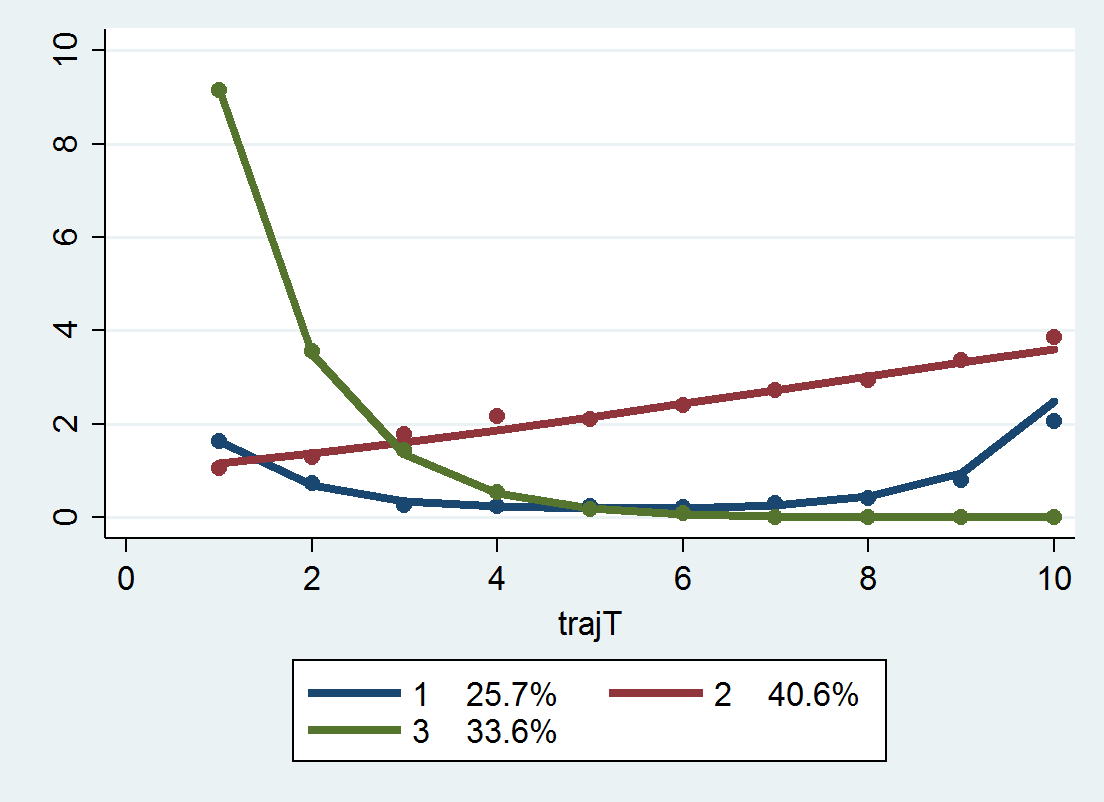Now for absolute model fit statistics, there are the average posterior probabilities, the odds of correct classification, and the observed classification proportion versus the expected classification proportion. Here I made a program that I will surely be ashamed of later (I should not brutalize the data and do all the calculations in matrix), but it works and produces an ugly table to get us these stats.

``````*I made a function to print out summary stats
program summary_table_procTraj
preserve
*updating code to drop missing assigned observations
drop if missing(_traj_Group)
*now lets look at the average posterior probability
gen Mp = 0
foreach i of varlist _traj_ProbG* {
replace Mp = `i' if `i' > Mp
}
sort _traj_Group
*and the odds of correct classification
by _traj_Group: gen countG = _N
by _traj_Group: egen groupAPP = mean(Mp)
by _traj_Group: gen counter = _n
gen n = groupAPP/(1 - groupAPP)
gen p = countG/ _N
gen d = p/(1-p)
gen occ = n/d
*Estimated proportion for each group
scalar c = 0
gen TotProb = 0
foreach i of varlist _traj_ProbG* {
scalar c = c + 1
quietly summarize `i'
replace TotProb = r(sum)/ _N if _traj_Group == c
}
gen d_pp = TotProb/(1 - TotProb)
gen occ_pp = n/d_pp
*This displays the group number [_traj_~p],
*the count per group (based on the max post prob), [countG]
*the average posterior probability for each group, [groupAPP]
*the odds of correct classification (based on the max post prob group assignment), [occ]
*the odds of correct classification (based on the weighted post. prob), [occ_pp]
*and the observed probability of groups versus the probability [p]
*based on the posterior probabilities [TotProb]
list _traj_Group countG groupAPP occ occ_pp p TotProb if counter == 1
restore
end``````

This should work after any model as long as the naming conventions for the assigned groups are `_traj_Group` and the posterior probabilities are in the variables `_traj_ProbG*`. So when you run

``summary_table_procTraj``

You get this ugly table:

```     | _traj_~p   countG   groupAPP        occ     occ_pp       p    TotProb |
|-----------------------------------------------------------------------|
1. |        1      103   .9379318   43.57342   43.60432   .2575   .2573645 |
104. |        2      136   .9607258   47.48513   48.30997     .34   .3361462 |
240. |        3      161   .9935605   229.0413   225.2792   .4025   .4064893 |```

The `groupAPP` are the average posterior probabilities – here you can see they are all quite high. `occ` is the odds of correct classification, and again they are all quite high. Update: Jeff Ward stated that I should be using the weighted posterior proportions for the OCC calculation, not the proportions based on the max. post. probability (that I should be using `TotProb` in the above table instead of `p`). So I have updated to include an additional column, `occ_pp` based on that suggestion. I will leave `occ` in though just to keep a paper trail of my mistake.

`p` is the proportion in each group based on the assignments for the maximum posterior probability, and the `TotProb` are the expected number based on the sums of the posterior probabilities. `TotProb` should be the same as in the Group Membership part at the bottom of the `traj` model. They are close (to 5 decimals), but not exactly the same (and I do not know why that is the case).

Next, to generate a plot of the individual trajectories, I want to reshape the data to long format. I use `preserve` in case I want to go back to wide format later and estimate more models. If you need to look to see how the `reshape` command works, type `help reshape` at the Stata prompt. (Ditto for help on all Stata commands.)

``````preserve
reshape long count_ t_, i(id)``````

To get the behavior I want in the plot, I use a scatter plot but have them connected via `c(L)`. Then I create small multiples for each trajectory group using the `by()` option. Before that I slightly jitter the count data, so the lines are not always perfectly overlapped. I make the lines thin and grey — I would also use transparency but Stata graphs do not support this.

``````gen count_jit = count_ + ( 0.2*runiform()-0.1 )
graph twoway scatter count_jit t_, c(L) by(_traj_Group) msize(tiny) mcolor(gray) lwidth(vthin) lcolor(gray)``````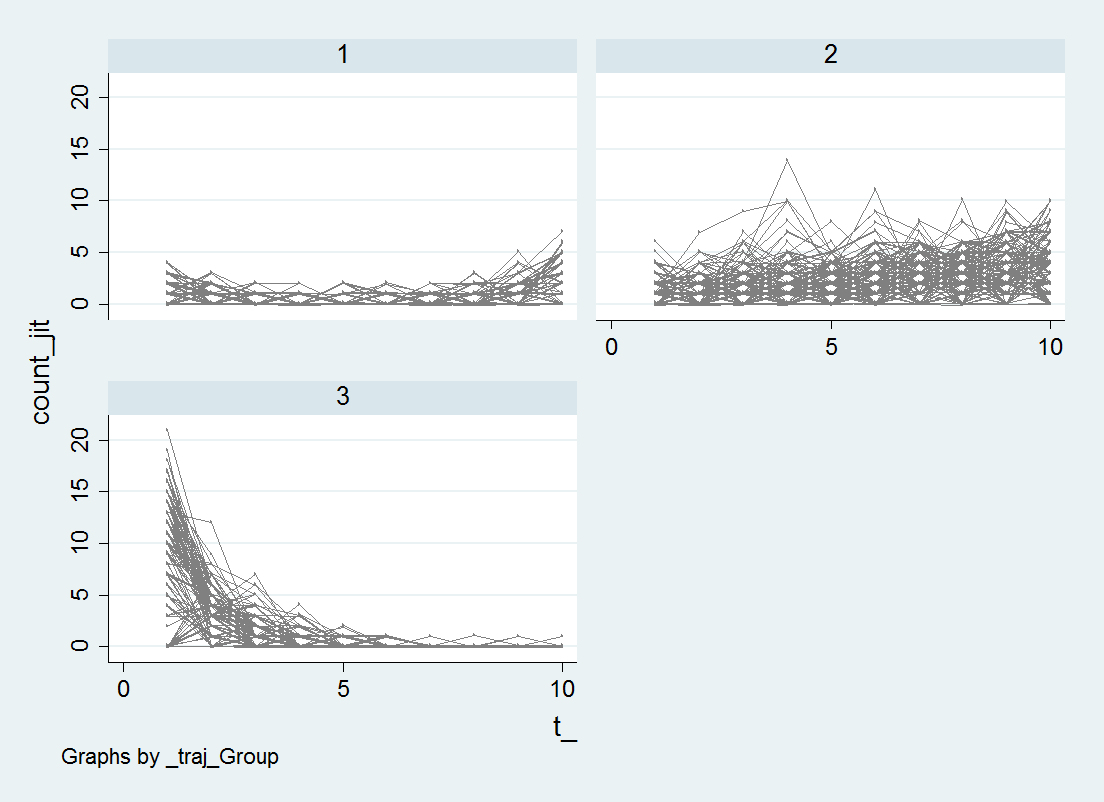I’m too lazy at the moment to clean up the axis titles and such, but I think this plot of the individual trajectories should always be done. See Breaking Bad: Two Decades of Life-Course Data Analysis in Criminology, Developmental Psychology, and Beyond (Erosheva et al., 2014).

While this fit looks good, this is not the correct number of groups given how I simulated the data. I will give those trying to find the right answer a few hints; none of the groups have a higher polynomial than 2, and there is a constant zero inflation for the entire sample, so `iorder(0)` will be the correct specification for the zero inflation part. If you take a stab at it let me know, I will fill you in on how I generated the simulation.